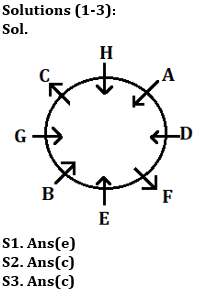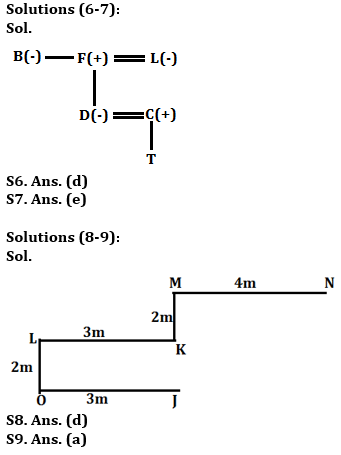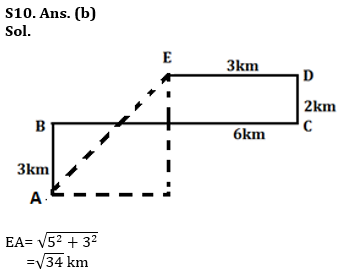Latest Banking jobs   »

# Reasoning Ability Quiz For Bank Foundation 2023 -02nd May

Directions (1-3): Study the information carefully and answer the questions given below.
Eight persons are sitting in a circular table in such a way that some are facing towards center and some are facing outside the center. D sits second to the right of E who faces towards the center. B sits third to the left of D. C sits opposite to F. One person sits between H and G. H and C sits immediate right to each other’s. H does not sit opposite to the one who sits immediate left of A. A faces towards center. B and G face same direction to H. F face same direction to C. C does not sit immediate left of E.

Q1. Who among the following sits second to the left of A?
(a) D
(b) G
(c) B
(d) H
(e) None of these

Q2. How many persons sit between C and E, when counted to the left of C?
(a) Three
(b) One
(c) Two
(d) Four
(e) None

Q3. How is B related to H?
(a) Second to the left
(b) Immediate right
(c) Third to the right
(d) Fourth to the left
(e) None of these

Directions (4-5): In these questions, relationship between different elements is show in the statements. The statements are followed by conclusions. Study the conclusions based on the given statements and select the appropriate answer:
(a) If only conclusion I follows.
(b) If only conclusion II follows.
(c) If either conclusion I or II follows
(d) If neither conclusion I nor II follows.
(e) If both conclusions I and II follow.

Q4. Statements: A≤M<T≤B=N>R>Y≥P>L
Conclusion I: M≤N II: L<B

Q5. Statements: K≥V=E≥M=X≤W>L≥S
Conclusion I: K≥X II: W>V

Directions (6-7): Study the following information carefully and answer the questions given below:
Six family members namely B, C, D, F, L and T are living and there are two married couples in the family. F is the father-in-law of C who is the father of T. B is the sister-in-law of L who is the mother of D.

Q6. What is the relation of B with respect to D?
(a) Niece
(b) Sister
(c) Mother
(d) Aunt
(e) None of these

Q7. How many males members are in the family?
(a) Two
(b) Three
(c) Four
(d) Either (a) or (c)
(e) Can’t be determined

Directions (8-9): Study the following information carefully to answer the given questions below.
Point L is 3m to the west of point K. Point M is 2m north of point K. Point N is 4m east of point M. Point O is 2m south of point L. Point J is 3m east of point O.

Q8. Which among the following points are in a straight line?
(a) O, J, K
(b) M, N, L
(c) L, K, J
(d) M, K, J
(e) L, K, M, N

Q9. In which direction is point N with respect to point O?
(a) North-east
(b) South-west
(c) North-west
(d) South
(e) None of these

Directions (10): Study the following information carefully and answer the given questions.
Shyam starts travelling in north direction from point A. After travelling 3km he reached point B. From there he takes a right turn and travel 6km. Then he reached point C and from there he turned towards left and walk 2km and reached point D. From there he turned towards left and after walking 3km, he finally stops at point E.

Q10. What is the shortest distance between point E and point A?
(a) √32
(b) √34
(c) 3√2
(d) 2√3
(e) None of these

Directions (11-15): Study the following alphanumeric series carefully and answer the questions given below:

A 1 & U V # F 3 S * 7 B \$ G 2 * 8 M 3 2 D 8 % N 2 4 5 H @ 5 Y 5

Q11. How many such numbers are there which is preceded by alphabets and followed by symbols?
(a) One
(b) Two
(c) Three
(d) Four
(e) None

Q12. Which of the following element is 21st from right end of the series?
(a) \$
(b) G
(c) 2
(d) 7
(e) None of these

Q13. If all symbols are eliminated from the series, then, which of the following element is 15th from the left end of the series?
(a) 3
(b) D
(c) 8
(d) 2
(e) None of these

Q14. How many such symbols are there which is immediately preceded by numbers and followed by vowels?
(a) Two
(b) None
(c) One
(d) Four
(e) Three

Q15. If all numbers are eliminated from the series, then, which of the following element is 13th from the left end of the series?
(a) M
(b) N
(c) *
(d) @
(e) None of these

SolutionsSolutions (4-5):
S4. Ans(b)
Sol. I: M≤N(False) II: L<B(True)

S5. Ans(a)
Sol. I: K≥X (True) II: W>V (False)Solutions (11-15):
S11. Ans(c)
S12. Ans(e)
S13. Ans(d)
S14. Ans(c)
S15. Ans(a)## FAQs

### What is the selection process of the Bank Clerk?

The selection process of the Bank Clerk is Prelims & Mains.

#### Congratulations!Union Budget 2023-24: Free PDF